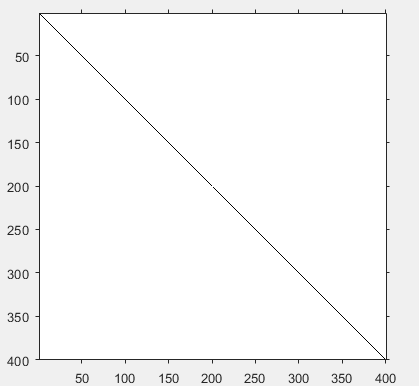# Plot a line along 2 points in MATLAB

Our objective is to plot a line along 2 points in MATLAB without using inbuilt functions for plotting. A black and white image can be represented as a 2 order matrix. The first order is for the rows and the second order is for the columns, the pixel value will determine the color of the pixel based on the grayscale color format.

Approach :

• We are given with 2 points. Let the coordinates of the points be (x1, y1) and (x2, y2).
• We find the slope of these 2 points and store it in m.
`m = (y2-y1)/(x2-x1);`
• Now for every pixel, find the slope between the pixel and one of the point.
`m2 = (y2-j)/(x2-i);`
• If the slope obtained is equal to the slope of the points, then change the color to black (0).

Implementation:

 `% MATLAB code to plot line through 2 points ` ` `  `% create a white image of size 400X400 ` `im = uint8(zeros(400, 400)) + 255; ` ` `  `% coordinates of point 1  ` `x1 = 100; ` `y1 = 100; ` ` `  `% coordinates of point 2 ` `x2 = 200; ` `y2 = 200; ` ` `  `% slope of points 1 and 2 ` `m = (y2-y1)/(x2-x1); ` ` `  `% accessing every pixel ` `for` `i = 1:400 ` `    ``for` `j = 1:400 ` `        ``m2 = (y2-j)/(x2-i); ` `        ``if` `m == m2 ` `            ``im(i, j) = 0; ` `        ``end` `    ``end`  `end` ` `  `% display the image ` `imshow(im); `

Output :My Personal Notes arrow_drop_upCheck out this Author's contributed articles.

If you like GeeksforGeeks and would like to contribute, you can also write an article using contribute.geeksforgeeks.org or mail your article to contribute@geeksforgeeks.org. See your article appearing on the GeeksforGeeks main page and help other Geeks.

Please Improve this article if you find anything incorrect by clicking on the "Improve Article" button below.

Article Tags :

Be the First to upvote.

Please write to us at contribute@geeksforgeeks.org to report any issue with the above content.